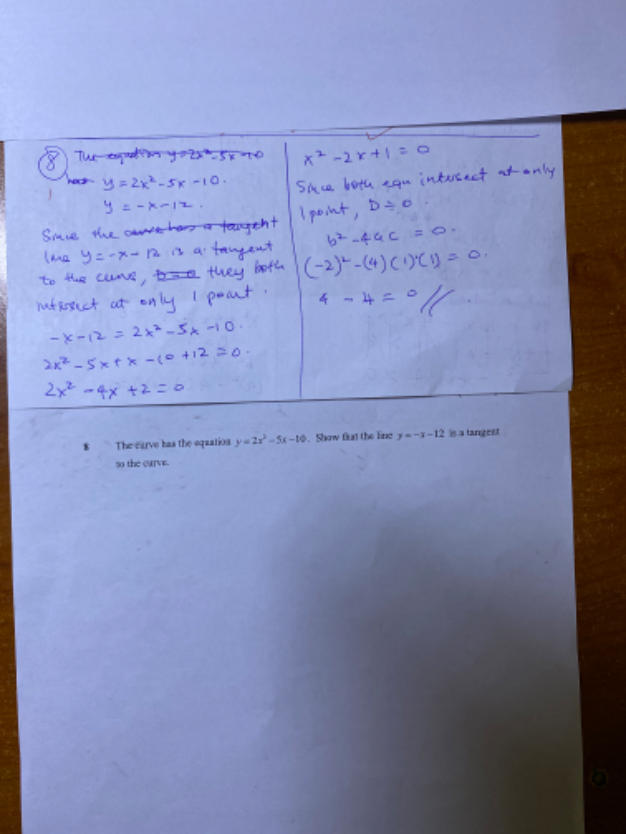# QuestionHi sushi 88, can I solve the ‘show’ question like this in the picture? Thanks

To show that the line is tangent to the quadratic function,

1. they only meet at one point of intersection
2. If the discriminant of the resulting quadratic is zero, then it is confirmed that the line is tangent to the parabola.

For intersection,  you have to equate the two expressions but how many points of intersections is still not clear at this stage, so you have to write:

To find points of intersection,

2x2 -5x – 10  = -x -12

………………… your working is correct here

x2 -2x + 1 = 0 (resulting quadratic)

(x-1)(x-1) = 0    => x = 1 , y = -13

Hence  (1,-13) is the only point of intersection.

Next, you can write to determine if b2 – 4ac = 0  for x2 -2x + 1 = 0 ( resulting quadratic)  to confirm the line is tangent to the curve,

Since  a = 1, b = -2, c = 1 =>  (-2)2 – 4(1)(1) = 4 – 4 = 0

Hence line y = -x -12 is tangent to the curve y =  2x2 -5x – 10